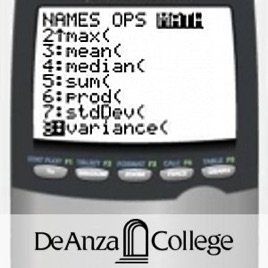12 episodes

Introduction to data analysis making use of graphical and numerical techniques to study patterns and departures from patterns. The student studies randomness with an emphasis on understanding variation, collects information in the face of uncertainty, checks distributional assumptions, tests hypotheses, uses probability as a tool for anticipating what the distribution of data may look like under a set of assumptions, and uses appropriate statistical models to draw conclusions from data. The course introduces the student to applications in engineering, business, economics, medicine, education, the sciences, and other related fields. The use of technology (computers or graphing calculators) will be required in certain applications.# Math 10: Elementary Statistics and Probability - With Captions De Anza College

• Education

Introduction to data analysis making use of graphical and numerical techniques to study patterns and departures from patterns. The student studies randomness with an emphasis on understanding variation, collects information in the face of uncertainty, checks distributional assumptions, tests hypotheses, uses probability as a tool for anticipating what the distribution of data may look like under a set of assumptions, and uses appropriate statistical models to draw conclusions from data. The course introduces the student to applications in engineering, business, economics, medicine, education, the sciences, and other related fields. The use of technology (computers or graphing calculators) will be required in certain applications.

• video
Chap01c - Sampling & Data

## Chap01c - Sampling & Data

• 28 min
• video
Chap02c - Descriptive Statistics

## Chap02c - Descriptive Statistics

• 27 min
• video
Chap03c - Probability Statistics

## Chap03c - Probability Statistics

• 28 min
• video
Chap04c - Discrete Random Variables

## Chap04c - Discrete Random Variables

• 26 min
• video
Chap05c - Continuous Random Variables

## Chap05c - Continuous Random Variables

• 27 min
• video
Chap06c - The Normal Distribution

• 26 min